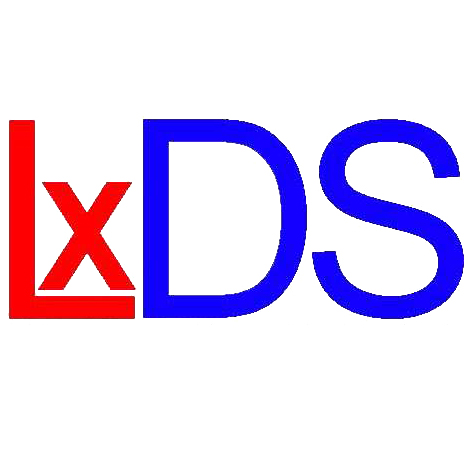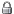LISBON DYNAMICAL SYSTEMS GROUP
 LxDS People Research Seminars Events Opportunities

## 7th ONE DAY MEETING ON DYNAMICAL SYSTEMS

### PROGRAM

 14:30 - 15:30 Vanessa Ramos (CMUP, PT, UFMA, BRAZIL) Equilibrium States for Hyperbolic Potentials Let $f\colon M \to M$ be a continuous map defined on a compact metric space $M$ and let $\phi\colon M \to \mathbb{R}$ be a real continuous function. In this classical setting, we say that $\mu_\phi$ is an equilibrium state associated to $(f,\phi)$, if $\mu_\phi$ is an $f$-invariant probability measure characterized by the following variational principle: $$P_f(\phi) = h_{\mu_\phi}(f) + \int\phi\,d\mu_\phi = \sup_{\mu\in \mathcal{M}_f(M)}\left\{h_\mu(f) + \int\phi\,d\mu\right\}$$ where $P_f(\phi)$ denotes the topological pressure, $h_\mu(f)$ is the metric entropy and the supremum is taken over all $f$-invariants probabilities measures. Existence is a relatively soft property that can often be established via compactness arguments. Uniqueness is usually more subtle and requires a better understanding of the dynamics. In this talk, we will show uniqueness of equilibrium states associated to local diffeomorphisms $f\colon M \to M$ and hyperbolic Holder continuous functions $\phi\colon M \to \mathbb{R}$. 16:00 - 17:00 Jaqueline Siqueira (CMUP, PT, UFF, BRAZIL) On equilibrium states for impulsive semiflows. Impulsive dynamical systems may be interpreted as suitable mathematical models of real world phenomena that display abrupt changes in their behavior, and are described by three objects: a continuous semiflow on a metric space $X$; a set $D$ contained in $X$; where the flow experiments sudden perturbations; and an impulsive function $I\colon D \to X$; which determines the change on a trajectory each time it collides with the impulsive set $D$. We consider impulsive semiflows defined on compact metric spaces and give suficient conditions, both on the semiflows and the potentials, for the existence and uniqueness of equilibrium states. We also generalize the classical notion of topological pressure to our setting of discontinuous semiflows and prove a variational principle. This is a joint work with José Ferreira Alves and Maria de Fátima de Carvalho.

#### No registration required

For further informations please send an email to .

#### VENUE

Campo Grande, Edifício C6, Piso 2
P-1749-016 Lisboa

#### ORGANIZERS

João Lopes Dias (ISEG, CEMAPRE)
Pedro Duarte (FCUL, CMAF)
José Pedro Gaivão (ISEG, CEMAPRE)

Local information | PrivateFriday, July 01, 2022